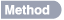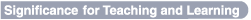STUDENTS’ MATHEMATICAL CONJECTURES AND JUSTIFICATIONSGlenda Anthony and Margaret Walshaw

 A pervasive theme emerging from mathematics education discussions in recent years is the issue of students’ active engagement with mathematical ideas. This theme also focuses on establishing inquiry-based classrooms that allow students to communicate their ideas. Communication is looked at closely in this study in relation to commutative and identity rules in mathematics.Data were collected from random samples of 50 Year 4 and 50 Year 8 students on five NEMP tasks. These tasks required students to justify their responses verbally and by manipulating cubes to illustrate commutative and identity rules in mathematics.• Is 4 plus 3 the same as 3 plus 4? Students were reasonably confident about the commutative property under addition. However, Year 8 students were noticeably more precise and more convincing in their explanations. Many Year 4 students were unable to provide a model to justify their conjecture. • What about 4 minus 3 and 3 minus 4? Are they the same? Students appeared much less assured about the commutative property for subtraction. They took longer to answer, and many students altered their original standpoint. Many Year 4 students modelled the equation directly rather than operated on the number relationship. Most Year 8 students could demonstrate the importance of number order, but were more likely to change their conjecture as they talked through the demonstration. • Does 2 times 5 give the same answer as 5 times 2? Students were more comfortable with commutativity under multiplication than under subtraction and just slightly more than they had been under addition. While most could provide a model to represent the problem, the understandings of Year 4 students evolved from often ill-founded ideas and inappropriate explanations. Those for Year 8 students demonstrated a more advanced conceptual understanding. No student exhibited sophisticated multiplicative thinking through the use of an array structure. Commutativity of multiplication was often modelled by way of additive reasoning. • Is there a number you can add to or take away from this number  but the number still stays the same? Just over half of the students could identify zero as the identity for addition and subtraction and were able to provide some explanation. While most Year 8 students confidently discussed the identity for multiplication, very few Year 4 students could recognise and explain it. A small number of students at both year levels were familiar with the identity for division. • What about multiplying or dividing? Is there a number you can multiply (or times) this number by or divide it by, so that the number stays the same? Tell me what it is and how this works. Many students appeared to be unfamiliar with the task of justifying a conjecture using cubes. While many confidently offered a conjecture and could provide a verbal explanation for the problems, they were not at ease when asked to model their thinking.If students are to realise that learning and doing mathematics involves solving problems in ways that are meaningful, classroom instruction must encourage and support discussion and reflection on the mathematics structure of number from an early age. More specifically, in relation to the assessment tasks analysed in this project, when students genuinely understand arithmetical ideas and can explain and justify the properties that they are using as they carry out calculations, they have learned the foundations of concepts belonging to algebra.